# How to use NOW function in Excel to get current date and time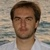by , updated on

In this tutorial, you will learn how to use a NOW formula in Excel to insert the current date and time as a dynamic value and how to make the NOW function static without auto update.

To insert the current date and time in your worksheet, Excel provides a special function named NOW. The function takes the information from your computer's system clock and updates automatically when the worksheet is opened or recalculated. The fact that this function takes no arguments may cause you to think that it leaves no room for customization. However, you can format the result exactly the way you need, so that a NOW formula shows only time, only date, or both. Find full details in the examples that follow.

## Excel NOW function

The NOW function in Excel returns the current date and time value.

The syntax is as simple as it could possibly be. No arguments are required:

NOW()

The NOW function is available in all versions of Excel 2007 through Excel 365.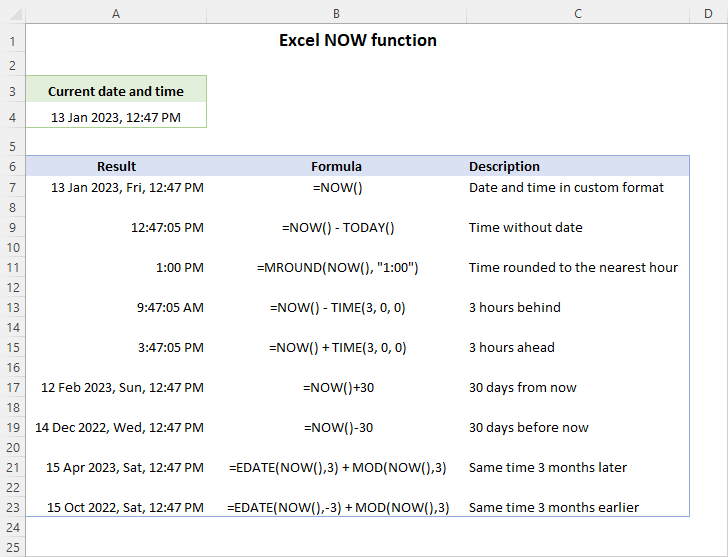### NOW function tips and notes

• The NOW function returns the current date and time in the form of a decimal number, where an integer represents the date, and a fractional value represents the time. For more information, see Excel time format.
• The date time value is returned in the default format set in your Region settings. This can be easily changed by applying a custom time format.
• The NOW function doesn't update continuously. Like any volatile function, it updates only when a workbook is opened or recalculated.
• To return only date without time, use the TODAY function instead of the NOW function. For more details, see NOW vs. TODAY.

## How to use NOW function in Excel

To insert a NOW formula in a worksheet, just type the equality sign, the function's name and empty parentheses:

`=NOW()`

Today's date and the current time will immediately appear in the cell in the default format. To have it displayed differently, apply a custom date time format as explained in the above linked tutorial.

### Get only time without date

To get the Excel NOW function to show time only, set a custom time format, for instance h:mm:ss. Please note, this will only change the visual representation. The underlying value in the cell will still have both parts - an integer representing the date and a fractional part representing the time.

Alternatively, you can subtract the date from the NOW() value, and then format the result to your liking.

`=NOW() - TODAY()`

This way, you'll get the current time without date. Unlike just formatting, the above formula completely removes the date part of the value stored in the cell. If you apply the General format, you will see that the integer representing the date is zero.

### Show only date without time

To make the NOW function to display date only, use any date format you want, for example mmmm d, yyyy.

The result will look like in the image below: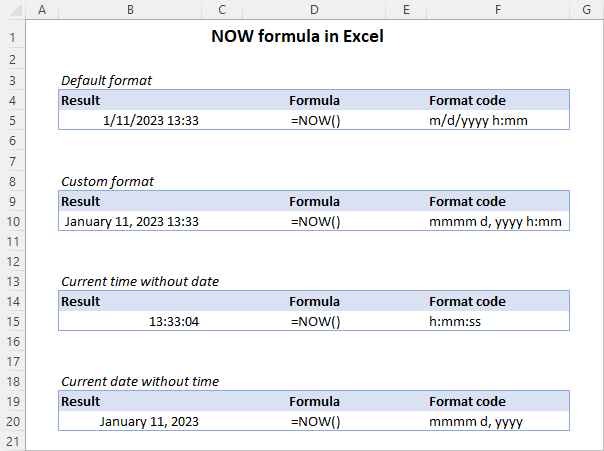## How to return same time on another day

To get the current time on another day, add or subtract a certain number of days to/from the NOW() value.

Here are a few examples of such NOW formulas:

Formula Description
=NOW() +7 Same time next week
=NOW() -7 Same time last week
=NOW() +30 Current time 30 days from now
=NOW() -30 Current time 30 days before now

To return the current time a few months from/before now, use the NOW function in combination with EDATE and MOD.

For example, to get the same time 3 months later, the formula is:

`=EDATE(NOW(), 3) + MOD(NOW(), 3)`

To return the current time 3 months earlier, the formula takes this form:

`=EDATE(NOW(), -3) + MOD(NOW(), 3)`

Here, you use the EDATE function to add or subtract the specified number of months to the current date - the result is the date value without time. To find time, you build a MOD formula that returns remainder after dividing the current date time value by the number of months, and then add up the results.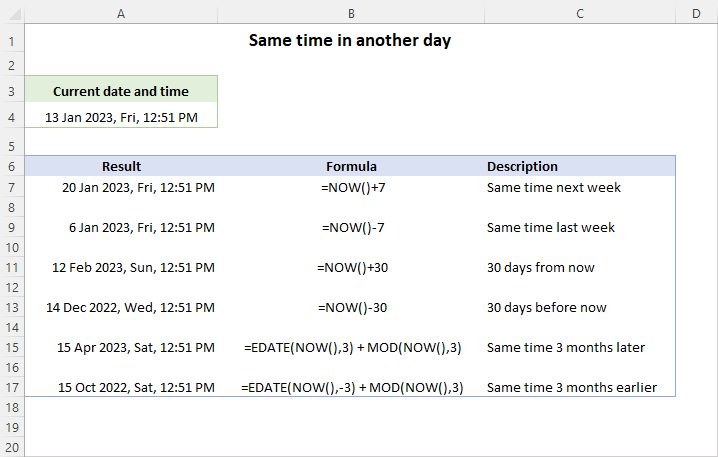## How to get current time rounded to the nearest hour

To round the current time to the nearest hour, use the MROUND function.

`=MROUND(NOW(), "1:00")`

This generic formula can be easily customized to handle more specific cases.

For instance, to return the current time 10 days from now rounded to nearest hour, the formula is:

`=MROUND(NOW() +10, "1:00")`

To get the current time 10 days before now rounded to nearest hour, use this formula:

`=MROUND(NOW() -10, "1:00")`

The results may look as follows: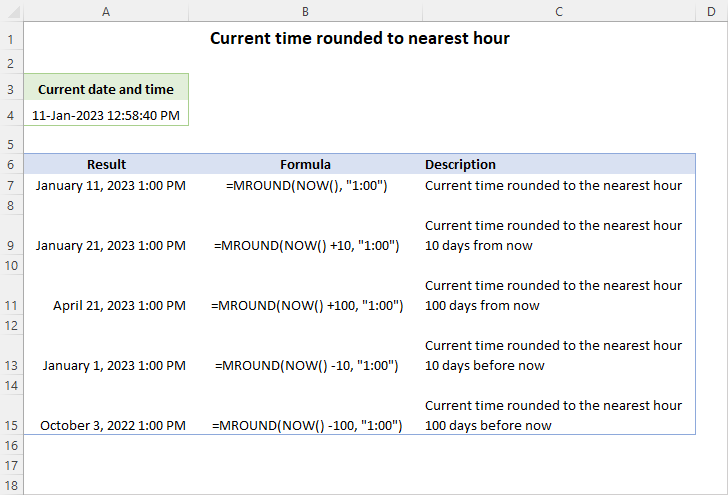## Add or subtract hours, minutes and seconds to current time

To add or subtract a certain amount of time from/to the current time, supply different time units with the help of the TIME function.

Let's say you are currently in the UTC+3 time zone. To get the Coordinated Universal Time (UTC), you need to subtract 3 hours from your local time, which can be easily done with this formula:

`=NOW() - TIME(3, 0, 0)`

And here's a slightly more complex case. To get the Indian Standard Time (IST), which is 2 hours and 30 minutes ahead of your local time, you provide the corresponding values for both the hour and minute argument of the TIME function:

`=NOW() + TIME(2, 30, 0)`

The image below shows a few more formula examples to calculate the current time in different time zones. To display only time without date, the custom number format h:mm:ss is applied to the results.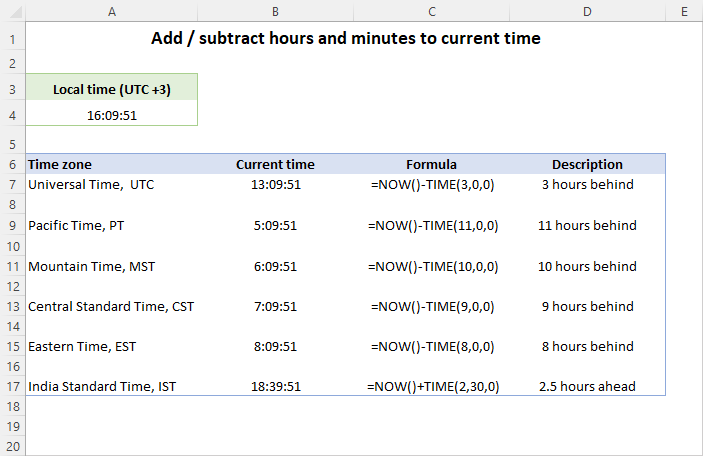## How to convert NOW to text in Excel

To turn the NOW() value into a text string in the desired format, embed it in the TEXT function like this:

TEXT(NOW(), format_code)

For example, to convert the current date and time into a text string such as "January 12, 2023 12:32 PM", the formula goes as follows:

`=TEXT(NOW(), "mmmm d, yyyy h:mm AM/PM")`

If needed, you can include some custom text or characters in the format code. For instance:

`=TEXT(NOW(), "mmmm d, yyyy at h:mm AM/PM")`

The result will appear as "January 12, 2023 at 12:37 PM".

More formula examples are shown in the screenshot below: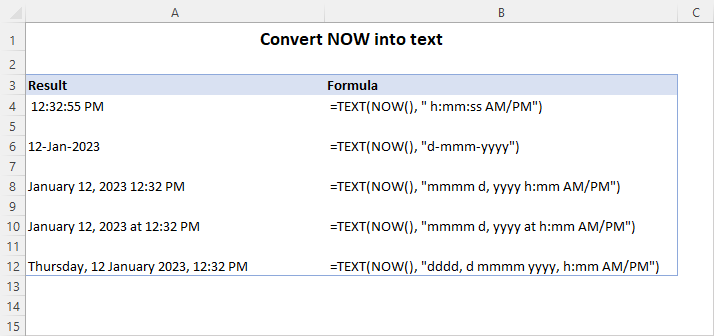## How to combine text with NOW function

There are 3 main methods to combine text with the NOW function in Excel:

### CONCATENATE and NOW

To join different text pieces with the date time value in the format of your choice, use the CONCATENATE function. The text values should be enclosed in double quotes, and NOW() is to be nested in TEXT as discussed in the previous example. For instance:

`=CONCATENATE("The current time is ", TEXT(NOW(), "h:mm:ss"))`

This formula works nicely in all versions of Excel.

### CONCAT and NOW

In Excel 2019 and later, you can use the CONCAT function for the same purpose. For example:

`=CONCAT("Today is ", TEXT(NOW(), "mmmm, d, yyyy"), CHAR(10), "Local time is ", TEXT(NOW(), "h:mm AM/PM"))`

Here, we additionally concatenate the CHAR(10) formula to have the date and time values displayed in two separate lines.

### "&" character and NOW

One more way to combine strings in Excel is the concatenation operator (&). It is supported in all Excel versions and can make a formula a bit more compact. For example:

`="Currently it's " & TEXT(NOW(), "h:mm:ss") & " here"`

And here are all three formulas in action: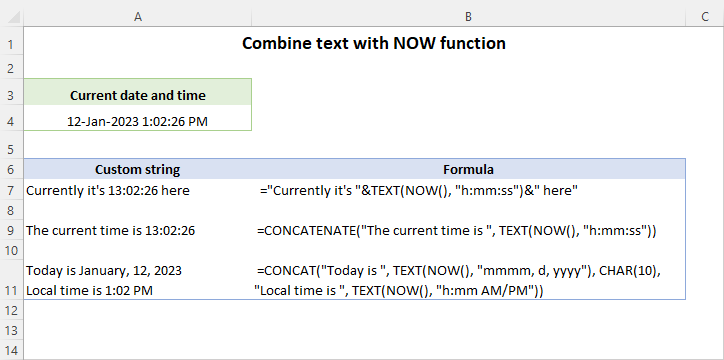## How to make Excel NOW function static

Under some circumstances, you may need to keep the value returned by the NOW function as-is without auto update. So, is there a way to stop the NOW() formula from updating every time the workbook is opened? The answer is no, it's not possible to fix the NOW function is Excel as it is designed to always return the current time.

What you can do is to create your own function that will return the current date and time as a static value. For this, add the following VBA code in your workbook. For the detailed instructions, you can refer to How to insert VBA code in Excel.

Like the native NOW function, our user-defined function does not take any arguments, just empty parentheses after the function name:

`=NowStatic()`

Once the formula is entered into a cell, it will return the current date and time as a static value that will never change. By default, the output appears as a decimal number in the General format. By applying a custom number format to the formula cell, you can get the result as a date time value, only date, or only time: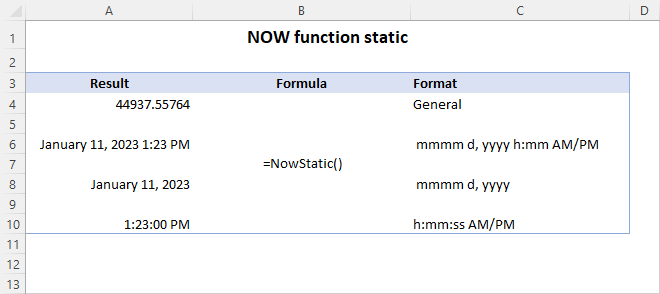Other options to insert the current date and time as an unchangeable value are:

## What is the difference between NOW and TODAY in Excel?

The TODAY and NOW functions are similar in that they both display the current date, are volatile, and take no arguments.

The difference between TODAY and NOW is:

• TODAY only returns the date value, which is stored as a whole number in the internal Excel system.
• NOW returns the date and time value, which includes the integer representing the date and a fractional value representing the time. A formula cell can be formatted to display only time, only date, or both. From this viewpoint, you can think of NOW as a more comprehensive version of TODAY.

## Excel NOW() function is not updating automatically

An important point to note is that the NOW function in Excel does not update continuously. It only refreshes when you open the workbook or when Excel recalculates it. If nothing has been changed on the sheet, there is nothing to recalculate, consequently NOW() won't be updated.

To get a NOW formula to update, you can use one of these shortcuts:

• F9 - recalculate all open workbooks.
• Shift + F9 - recalculate the active worksheet only.

Also, make sure that Calculation Options is set to Automatic in your Excel.

That's how to efficiently use the NOW function in Excel. I thank you for reading and hope to see you on our blog next week!